# Residual plots for Fit Binary Logistic Model

Find definitions and interpretation guidance for the residual plots.

## Histogram of residuals

The histogram of the deviance residuals shows the distribution of the residuals for all observations.

The interpretation of the plot is the same whether you use deviance residuals or Pearson residuals. When the model uses the logit link function, the distribution of the deviance residuals is closer to the distribution of residuals from a least squares regression model. The deviance residuals and the Pearson residuals become more similar as the number of trials for each combination of predictor settings increases.

### Interpretation

Use the histogram of the residuals to determine whether the data are skewed or include outliers. The patterns in the following table may indicate that the model does not meet the model assumptions. When the model does not meet the assumptions, the normal approximation confidence intervals for predictions are probably inaccurate.
Pattern What the pattern may indicate
A long tail in one direction Skewness
A bar that is far away from the other bars An outlier

Because the appearance of a histogram depends on the number of intervals used to group the data, don't use a histogram to assess the normality of the residuals. Instead, use a normal probability plot.

## Normal probability plot of residuals

The normal probability plot of the residuals displays the residuals versus their expected values when the distribution is normal.

The interpretation of the plot is the same whether you use deviance residuals or Pearson residuals. When the model uses the logit link function, the distribution of the deviance residuals is closer to the distribution of residuals from a least squares regression model. The deviance residuals and the Pearson residuals become more similar as the number of trials for each combination of predictor settings increases.

### Interpretation

Use the normal probability plot of the residuals to verify the assumption that the residuals are normally distributed. The normal probability plot of the residuals should approximately follow a straight line.

The following patterns violate the assumption that the residuals are normally distributed.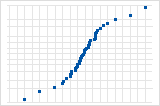S-curve implies a distribution with long tails.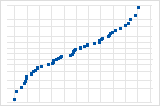Inverted S-curve implies a distribution with short tails.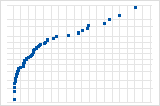Downward curve implies a right-skewed distribution.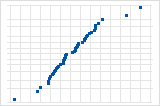A few points lying away from the line implies a distribution with outliers.

If you see a nonnormal pattern, use the other residual plots to check for other problems with the model, such as missing terms or a time order effect. If the residuals do not follow a normal distribution, the normal approximation confidence intervals and Wald test p-values can be inaccurate.

## Residuals versus fits

The residuals versus fits graph plots the residuals on the y-axis and the logit of fits on the x-axis.
###### Note

In binary logistic regression, Minitab does not provide this plot when the data are Binary Response/Frequency format (single trial per row).

The interpretation of the plot is the same whether you use deviance residuals or Pearson residuals. When the model uses the logit link function, the distribution of the deviance residuals is closer to the distribution of residuals from a least squares regression model. The deviance residuals and the Pearson residuals become more similar as the number of trials for each combination of predictor settings increases.

### Interpretation

Use the residuals versus fits plot to verify the assumption that the residuals are randomly distributed. Ideally, the points should fall randomly on both sides of 0, with no recognizable patterns in the points.

The patterns in the following table may indicate that the model does not meet the model assumptions.
Pattern What the pattern may indicate
Fanning or uneven spreading of residuals across fitted values An inappropriate link function
Curvilinear A missing higher-order term or an inappropriate link function
A point that is far away from zero An outlier
A point that is far away from the other points in the x-direction An influential point
The following graphs show an outlier and a violation of the assumption that the variance of the residuals is constant.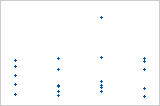Plot with outlierOne of the points is much larger than all of the other points. Therefore, the point is an outlier. If there are too many outliers, the model may not be acceptable. You should try to identify the cause of any outlier. Correct any data entry or measurement errors. Consider removing data values that are associated with abnormal, one-time events (special causes). Then, repeat the analysis.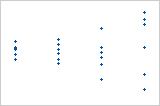Plot with nonconstant varianceThe variance of the residuals increases with the fitted values. Notice that, as the value of the fits increases, the scatter among the residuals widens. This pattern indicates that the variances of the residuals are unequal (nonconstant).
If you identify any patterns or outliers in your residual versus fits plot, consider the following solutions:
Issue Possible solution
Nonconstant variance Consider using different terms in the model, a different link function, or weights.
An outlier or influential point
1. Verify that the observation is not a measurement error or data-entry error.
2. Consider performing the analysis without this observation to determine how it impacts your results.

## Residuals versus order

The residuals versus order plot displays the residuals in the order that the data were collected.

The interpretation of the plot is the same whether you use deviance residuals or Pearson residuals. When the model uses the logit link function, the distribution of the deviance residuals is closer to the distribution of residuals from a least squares regression model. The deviance residuals and the Pearson residuals become more similar as the number of trials for each combination of predictor settings increases.

### Interpretation

Use the residuals versus order plot to verify the assumption that the residuals are independent from one another. Independent residuals show no trends or patterns when displayed in time order. Patterns in the points may indicate that residuals near each other may be correlated, and thus, not independent. Ideally, the residuals on the plot should fall randomly around the center line:If you see a pattern, investigate the cause. The following types of patterns may indicate that the residuals are dependent.

## Residuals versus the variables

The residuals versus variables plot displays the residuals versus another variable. The variable could already be included in your model. Or, the variable may not be in the model, but you suspect it affects the response.

The interpretation of the plot is the same whether you use deviance residuals or Pearson residuals. When the model uses the logit link function, the distribution of the deviance residuals is closer to the distribution of residuals from a least squares regression model. The deviance residuals and the Pearson residuals become more similar as the number of trials for each combination of predictor settings increases.

### Interpretation

If the variable is already included in the model, use the plot to determine whether you should add a higher-order term of the variable. If the variable is not already included in the model, use the plot to determine whether the variable is affecting the response in a systematic way.

These patterns can identify an important variable or term.
Pattern What the pattern may indicate
Pattern in residuals The variable affects the response in a systematic way. If the variable is not in your model, include a term for that variable and refit the model.
Curvature in the points A higher-order term of the variable should be included in the model. For example, a curved pattern indicates that you should add a squared term.
By using this site you agree to the use of cookies for analytics and personalized content.  Read our policy